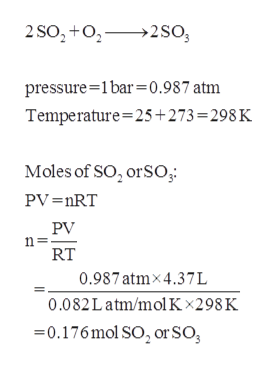# Calculate the amount of energy in the form of heat that is produced when a volume of 4.37 L of SO2(g) is converted to 4.37 L of SO3(g) according to this process at constant pressure and temperature of 1.00 bar and 25.0 ° C. Assume ideal gas behavior.

Question
1 views

Calculate the amount of energy in the form of heat that is produced when a volume of 4.37 L of SO2(g) is converted to 4.37 L of SO3(g) according to this process at constant pressure and temperature of 1.00 bar and 25.0 ° C. Assume ideal gas behavior.

check_circle

star
star
star
star
star
1 Rating
Step 1

Given, 4.37L of SO2(g) converted to 4.37L of SO3(g).

Pressure = 1.00bar.

Temperature = 25.0 degree Celsius.

Step 2

The balanced reaction and the moles of SO2(g) and S...help_outlineImage Transcriptionclose2 SO,+o 2So pressure 1bar=0.987 atm Temperature 25+273 = 298 K Moles of SO, orSO; PV =nRT PV RT 0.987atmx4.37L 0.082Latm/molKx298K -0.176mol SO2 or SO fullscreen

### Want to see the full answer?

See Solution

#### Want to see this answer and more?

Solutions are written by subject experts who are available 24/7. Questions are typically answered within 1 hour.*

See Solution
*Response times may vary by subject and question.
Tagged in

### Physical Chemistry